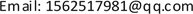OEOptoelectronics2164-5450Scientific Research Publishing10.12677/OE.2019.91009OE-29445OE20190100000_48926883.pdf工程技术 大口径拼接镜平移误差对其成像质量影响研究 Study on the Influence of Translation Error of Large Aperture Stitching Mirror on Its Imaging Quality 雪微1*长春理工大学理学院，吉林 长春2801201909015661© Copyright 2014 by authors and Scientific Research Publishing Inc. 2014This work is licensed under the Creative Commons Attribution International License (CC BY). http://creativecommons.org/licenses/by/4.0/Copyright © 2019 by author(s) and Hans Publishers Inc.1. 引言

2. 平移误差对拼接镜成像质量影响理论分析

U ( x , y ) = A ( x , y ) [ c i r c x − B 2 D 2 ∗ e i ϕ 1 + c i r c x + B 2 D 2 ∗ e i ϕ 2 ] (1)

A ( x , y ) = { 1 , 子 孔 内 径 0 , 子 孔 径 外 (2)

Δ ϕ = ϕ 2 − ϕ 1 = 2 π λ p (3)

P S F ( u , v , λ ) = | F T [ U ( x , y ) ] | 2 = 2 D 2 / 2 J 1 2 ( π D u 2 + v 2 ) u 2 + v 2 (4)

P S F ( x , y , λ ) = 2 D 2 / 2 f 2 λ 2 J 1 2 ( π D / λ f x 2 + y 2 ) x 2 + y 2 [ 1 + cos ( 2 π p λ − 2 π B λ f x ) ] (5)

P S F t ( u , v , λ ) = ∑ k = 1 n P S F ( u , v , λ k ) Δ λ n (6)

3. 平移误差对拼接镜成像质量影响仿真分析

4. 结论